Open in App
Not now

# Select Rows With Multiple Filters in Pandas

• Last Updated : 24 Jan, 2021

In this article, we are going to select rows using multiple filters in pandas. We will select multiple rows in pandas using multiple conditions, logical operators and using loc() function.

Selecting rows with logical operators i.e. AND and OR can be achieved easily with a combination of >, <, <=, >= and == to extract rows with multiple filters. loc() is primarily label based, but may also be used with a boolean array to access a group of rows and columns by label or a boolean array.

Dataset Used:

Creating a dataframe with columns Name, Class, Marks in English, Marks in Maths, and Marks in History. We are going to use the below dataset for all operations:

## Python

 `import` `pandas as pd`` ` `# initialize list of lists``data ``=` `[[``'John'``, ``8``, ``7``, ``6``, ``5``], [``'Paul'``, ``8``, ``3``, ``6``, ``4``],``        ``[``'Juli'``, ``9``, ``10``, ``9``, ``9``], [``'Geeta'``, ``9``, ``5``, ``4``, ``4``]]`` ` `# Create the pandas DataFrame``df ``=` `pd.DataFrame(``    ``data, columns``=``[``'Name'``, ``'Class'``, ``'English'``, ``                   ``'Maths'``, ``'History'``])`` ` `# print dataframe.``print``(df)`

Output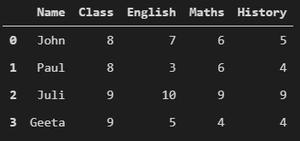Below are various operations which implement the selection of rows with multiple filters:

• Selecting row with students having marks is English greater than 6 and marks is maths greater than 8.

## Python

 `df1 ``=` `df[(df.English>``6``) & (df.Maths>``8``)]``print``(df1)`

Output: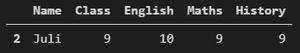• Selecting rows with students having marks in English greater than equal to 5 or marks is history greater than 7.

## Python

 `df1 ``=` `df[(df.English>``=``5``) | (df.History>``7``)]``print``(df1)`

Output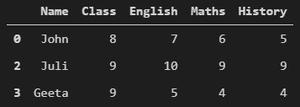• Selecting rows with students of class 9 having marks in English greater than equal to 5 or marks is history greater than 7.

## Python

 `df1 ``=` `df[(df.Class ``=``=` `9``) & ((df.English>``=``5``) | (df.History>``7``))]``print``(df1)`

Output: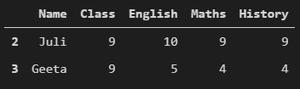• Selecting row with students having marks in English less than equal to 5 and marks is maths less than equal to 5 and marks is history less than equal to 5.

## Python

 `df1 ``=` `df[(df.English<``=``5``) & (df.Maths<``=``5``) & (df.History<``=``5``)]``print``(df1)`

Output: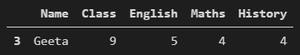• Selecting rows with students of class 8 having marks in English less than equal to 5 or marks is maths greater than 5 or marks is history less than equal to 5.

## Python

 `df1 ``=` `df[(df.Class ``=``=` `8``) & ((df.English<``=``5``) | (df.Maths>``5``) | (df.History<``=``5``))]``print``(df1)`

Output: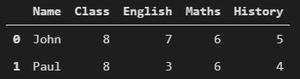• Selecting rows with loc() having marks in English greater than 6 and marks in maths greater than 6.

## Python

 `df1 ``=` `df.loc[(df[``'English'``]>``6``) & (df[``'Maths'``]>``6``)]``print``(df1)`

Output: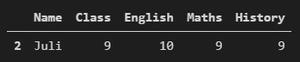• Selecting rows with loc() having students marks in English greater than 6 or marks in maths greater than 4. We only display columns with Name and Class.

## Python

 `df1 ``=` `df.loc[((df[``'English'``]>``6``) | (df[``'Maths'``]>``4``)),[``'Name'``,``'Class'``]]``print``(df1)`

Output: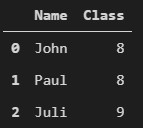My Personal Notes arrow_drop_up